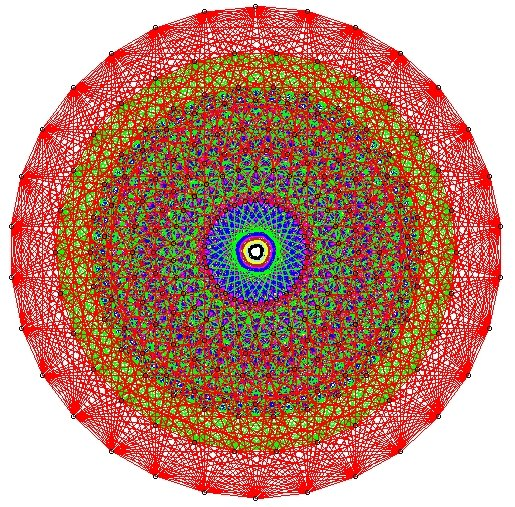Capabilities of the Atlas Software Here is a description of the main features of the software, Version 1.0 (February 2017). The groups: a general connected complex reductive group G; an inner class of strong real forms of G; a real form of G. Structure theory: the component group of G; the conjugacy classes of Cartan subgroups of G; the relative Weyl group of each Cartan subgroup; K-orbits on G/B and G/P Parabolic subgroups; real and theta-stable parabolics Representations: A parameter space {p|pϵS} for the standard and irreducible representations of G An arbitrary standard representation I(p) (pϵS) An arbitrary irreducible representation J(p) (the unique irreducible quotient of I(p)) The block B of J(p) The Kazhdan-Lusztig-Vogan polynomials of B The Character formula of any irreducible representation J(p) The composition series of any standard module I(p) The Jantzen filtration of I(p) Twisted versions all these objects (following Lusztig and Vogan) Vogan duality for B Branching to K: Parametrization of the irreducible representations of (possibly disconnected) K K-types of I(p) and J(p) Induction: Induction from an arbitrary representation on a real parabolic subgroup Cohomological induction in the weakly fair range Euler characteristic of cohomological induction in general Hermitian Forms: Signature of the c-invariant form on I(p) and J(p) Determine if J(p) admits an invariant Hermitian form Signature of invariant Hermitian form on J(p) Determine if the invariant Hermitian form on J(p) is definite, i.e. J(p) is unitary Here is some mathematical background.